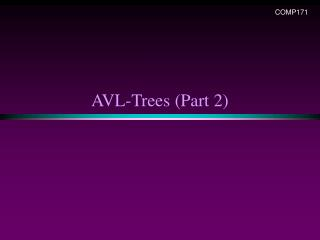Download PresentationAVL-Trees (Part 2)

# AVL-Trees (Part 2) - PowerPoint PPT PresentationDownload Presentation## AVL-Trees (Part 2)

- - - - - - - - - - - - - - - - - - - - - - - - - - - E N D - - - - - - - - - - - - - - - - - - - - - - - - - - -
##### Presentation Transcript

1. COMP171 AVL-Trees (Part 2)

2. A warm-up exercise … • Create a BST from a sequence, • A, B, C, D, E, F, G, H • Create a AVL tree for the same sequence.

3. More about Rotations When the AVL property is lost we can rebalance the tree via rotations • Single Right Rotation (SRR) • Performed when A is unbalanced to the left (the left subtree is 2 higher than the right subtree) and B is left-heavy (the left subtree of B is 1 higher than the right subtree of B). A B SRR at A B T3 T1 A T1 T2 T2 T3

4. Rotations • Single Left Rotation (SLR) • performed when A is unbalanced to the right (the right subtree is 2 higher than the left subtree) and B is right-heavy (the right subtree of B is 1 higher than the left subtree of B). A B SLR at A T1 B A T3 T2 T3 T1 T2

5. Rotations • Double Left Rotation (DLR) • Performed when C is unbalanced to the left (the left subtree is 2 higher than the right subtree), A is right-heavy (the right subtree of A is 1 higher than the left subtree of A) • Consists of a single left rotation at node A, followed by a single right at node C C C B SLR at A SRR at C A T4 B T4 A C T1 B A T3 T1 T2 T3 T4 A is balanced T2 T3 T1 T2 DLR = SLR + SRR Intermediate step, get B

6. Rotations • Double Right Rotation (DRR) • Performed when A is unbalanced to the right (the right subtree is 2 higher than the left subtree), C is left-heavy (the left subtree of C is 1 higher than the right subtree of C) • Consists of a single right rotation at node C, followed by a single left rotation at node A A A B SRR at C SLR at A T1 C T1 B A C B T4 T2 C T1 T2 T3 T4 T2 T3 T3 T4 DRR = SRR + SLR

7. Insertion Analysis logN • Insert the new key as a new leaf just as in ordinary binary search tree: O(logN) • Then trace the path from the new leaf towards the root, for each node x encountered: O(logN) • Check height difference: O(1) • If satisfies AVL property, proceed to next node: O(1) • If not, perform a rotation: O(1) • The insertion stops when • A single rotation is performed • Or, we’ve checked all nodes in the path • Time complexity for insertion O(logN)

8. class AVL { public: AVL(); AVL(const AVL& a); ~AVL(); bool empty() const; bool search(const double x); void insert(const double x); void remove(const double x); private: Struct Node { double element; Node* left; Node* right; Node* parent; Node(…) {…}; // constructuro for Node } Node* root; int height(Node* t) const; void insert(const double x, Node*& t) const; // recursive function void singleLeftRotation(Node*& k2); void singleRightRotation(Node*& k2); void doubleLeftRotation(Node*& k3); void doubleRightRotation(Node*& k3); void delete(…) } Implementation:

9. Deletion from AVL Tree • Delete a node x as in ordinary binary search tree • Note that the last (deepest) node in a tree deleted is a leaf or a node with one child • Then trace the path fromthe new leaf towards the root • For each node x encountered, check if heights of left(x) and right(x) differ by at most 1. • If yes, proceed to parent(x) • If no, perform an appropriate rotation at xContinue to trace the path until we reach the root

10. Deletion Example 1 20 20 15 35 10 35 25 10 18 40 25 5 15 40 30 38 45 18 30 38 45 50 50 Single Rotation Delete 5, Node 10 is unbalanced

11. Cont’d 35 20 15 35 20 40 25 10 18 40 38 15 45 25 30 38 45 50 10 18 30 50 Continue to check parents Oops!! Node 20 is unbalanced!! Single Rotation For deletion, after rotation, we need to continue tracing upward to see if AVL-tree property is violated at other node. Different from insertion!

12. Rotation in Deletion • The rotation strategies (single or double) we learned can be reused here • Except for one new case: two subtrees of y are of the same height rotate with left child rotate with right child

13. Deletion Example 2 Right most child of left subtree Double rotation

14. Example 2 Cont’d New case